# Kerala Syllabus 6th Standard Maths Solutions Chapter 10 Letter Math

## Kerala State Syllabus 6th Standard Maths Solutions Chapter 10 Letter Math

### Letter Math Text Book Questions and Answers

Addition and Subtraction Textbook Page No. 149

Mary is now 4 years old; and her brother Johny is 8.
What would be Mary’s age after 2 years?
And Johny’s age?
What were their ages 3 years ago?
Can you fill up the blanks in the table.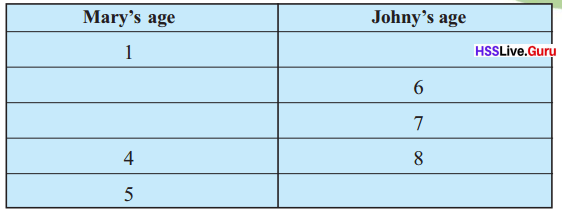In this, how do we compute Johny’s age from Mary’s age?
Adding 4 to Mary’s age gives Johny’s age, right?
We can shorten it like this:
Johny’s age = Mary’s age +4
There’s a trick to shorten it further. Let’s write m for Mary’s age and
j for Johny’s age. Then we can write
j = m + 4Explanation:
Difference between Johny’s age and Mary’s age = Johny’s age – Mary’s age
= 8 – 4
= 4.
Johny’s age = Difference between Johny’s age and Mary’s age + Mary’s age
=> 1 + 4
=> 5 years.
Johny’s age – Difference between Johny’s age and Mary’s age = Mary’s age
=> 6 – 4
=> 2 years.
Johny’s age – Difference between Johny’s age and Mary’s age = Mary’s age
=> 7 – 4
=> 3 years.
Johny’s age = Difference between Johny’s age and Mary’s age + Mary’s age
=> 5 + 4
=> 9 years.

Here, the letter m which stands for Mary’s age can be any of the numbers 1, 2, 3 and so on. Accordingly we get
the numbers 5, 6, 7 and so on as j.
Another problem:
See this figure;However we draw the slanted line, what is the relation connecting the angles on the left and right?
We can write it like this:

The sum of the angles on the left and right is 180°.
What if we write the measure of the angle on the left as l° and the measure of the angle on the right as r°, then we can shorten this as:
l + r = 180One fact different ways Textbook Page No. 150

We can say the same fact in different ways.

1. Johny is 4 years older than Mary.
2. Mary is 4 years younger than Johny.
3. The difference in ages between Johny and his younger sister Mary is 4.

When we write such relations using letters also, we can put them in different ways. If we write j for Johny’s age and m for Mary’s age, the statements above become

1. j = m + 4
2. m = j – 4
3. j – m = 4

In what all ways can we state the relationship between the angles a line makes on the two sides of
another line?
Can you write each of these using letters?7*

Now look at this figure: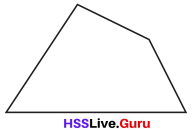A figure of four sides. Drawing a line from one corner to the opposite comer, we can split it into two triangles;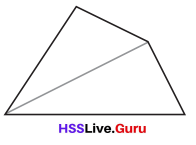What about a five sided figure?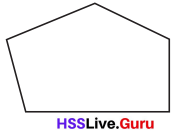We can draw lines from one comer to two other corners to get three triangles.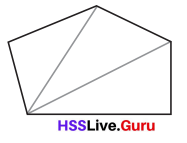And a six sided figure?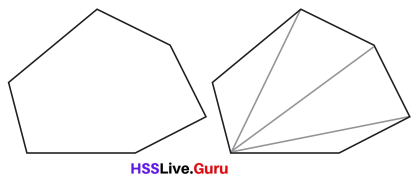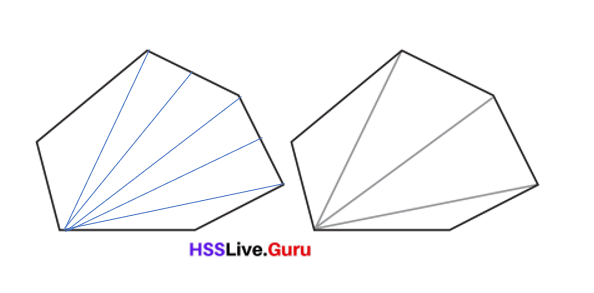Explanation:
A six sided figure can be drawn by joining the edges and making two straight lines in between the lines.Changing angles

We can use a slider to make an angle which can be changed as we like
Choose slider and click on the Graphic view. In the window which opens up, choose Integer and give Min = 0, Max = 180. Click Apply. We get a slider named n.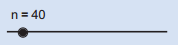Mark two points A, B.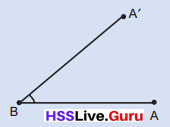Choose Angle with Given size and click on A and B in order. In the window which opens up, give n° as the size of the angle and click OK. We get a new point A’.
Draw the lines BA and BA’. As we move the slider, the size of angle B changes.

Draw seven sided and eight sided figures like this. From one particular comer, draw lines to other corners to split them into triangles. Make a table like this: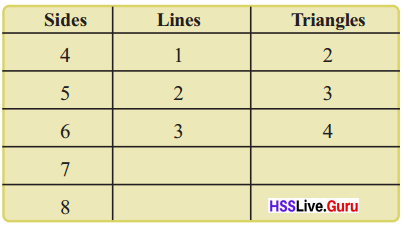Explanation:
Number of lines + 1 = Number of Triangles.
=> 3 + 1 = 4.
=> 4 + 1 = 5.
=> 5 + 1 = 6.

In a 12 sided figure, how many such lines can be drawn from one particular comer?
9 lines can be drawn from one particular comer.

Explanation:
Number of Sides – 3 = Number of lines
=> 12 – 3
=> 9.

• What is the relation connecting the number of sides and number of lines in general?
The relation connecting the number of sides and number of lines in general is they are directly connected to eachother.

Explanation:
The number of lines and number of sides are directly related to eachother in forming the number of shapes.

• What is the relation connecting the number of sides and the number of triangles?
The number of lines is the relation connecting the number of sides and the number of triangles.

Explanation:
The relation connecting the number of sides and the number of triangles is the number of lines used.

• What is the relation connecting the number of lines and the number of triangles?
Number of sides used to be formed is the relation connecting the number of lines and the number of triangles.

Explanation:
Number of sides connects the number of lines and the number of triangles.

Writing the number of sides as s, the number of lines as l and the number of triangles as t, how do we write these relations?
This is how Sneha wrote them:

• s – 3 = l
• t + 2 = s
• t – 1 = l

In what other ways can we write them?
Try!
Other ways can we write them are:
t = s – 2.
t = l + 1.

Explanation:
t + 2 = s
=> t = s – 2.
t – 1 = l
=> t = l + 1.Regular polygons Textbook Page No. 152

Polygons with equal sides and equal angles are called regular polygons.We can easily draw such figures using Geogebra. We use Regular polygon to do this.

Choose this and click on two positions. In the window, which opens up give the number of sides and click OK.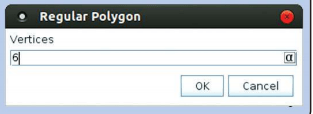Now look at this problem:

A shopkeeper decides to sell something for a price 1oo rupees more than what he bought it for. If the had bought it for 500 rupees, at what price would he sell it? What if the price at which he bought is 600 rupees? Here what is the relation between the prices at which he bought and the price at which he sells?

This hundred rupee increase in price is called the profit in the sale. If he wants a profit of 150 rupees, what would be the relation between how much the shopkeeper bought it for and how much he sells it for? What if he wants a profit of 200 rupees? Write down these relations using letters.

How do we write in general, the relation between the price at which a shopkeeper buys something, the price at which he sells it and the profit? Adding the profit to the price at which it is bought gives the price at which it is sold.
Writing the price at which it is bought as b, the profit as p and the price at which it is sold as s, we get
s = b + p

In what other ways can you write this relation?
In a transaction, the selling price is greater than the cost price, it means we earn a profit.

Explanation:
The selling price of it as S, the profit bought of it as profit P, the cost price for what it is purchased as C.
=> Profit = Selling price -Cost price.
=> P = SP – CP.

Question 1.
For getting books through post, 25 rupees has to be added to the price of the book, as postage. Fill in the blanks of the table below: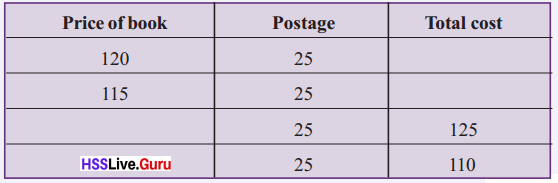In what all ways can we say the relation between the price of a book and the total cost? Write these using letters. What if the postage is 30 rupees? What if it is 35 rupees?Now if the postage also changes according to the price of the book, in what all ways can we say this relation?
Write them using letters.
Profit = Selling price – Cost price.

Explanation:
If the selling price(SP) is lesser than the cost price (CP), whatever difference you get between the two is the loss suffered. Similarly, Loss is C.P. – S.P. Always remember that you calculate profit or loss on the cost price.
Total cost (TC) = Price of the postage(CP) + Postage (P)
=> Total cost (TC) – Price of the postage(CP) = Postage (P)Question 2.
Make a table showing the number of girls, the number of boys and the total number of children
in each class of your school. What are the different ways of stating the relation between these numbers? Write these using letters.
C = G + B = 63.

Explanation:
Number of girls in a class (G)= 31.
Number of boys in a class (B) = 32.
Total number of students in class (C) = Number of girls in a class (G)+ Number of boys in a class (B)
= 31 + 32
= 63.

Question 3.
The sides of a triangle are 4 centimetres, 6 centimetres and 8 centimetres. What is its perimeter?
Denoting the length of the sides as a, b, c and the perimeter as p, how do we write the relation between them?
Perimeter of the triangle (p) = 18 cm.

Explanation:
Side of the triangle (a) = 4 cm.
Side of the triangle (b) = 6 cm.
Side of the triangle (c) = 8 cm.
Perimeter of the triangle (p) = Side of the triangle (a) + Side of the triangle (b) + Side of the triangle (c)
= 4 + 6 + 8
= 10 + 8
= 18 cm.Textbook Page No. 154

We can draw regular polygon with as many sides as we like, using a slider.
Choose slider and click. In the window which opens up, choose Integer and type 3 for min. We get a slider named n.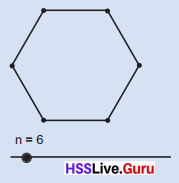Choose Regular polygon and click on two positions. In the window which opens up, give n as the number of sides instead of a specific number

Click and drag the dot on the slider to change the number n The number of sides of the polygon changes accordingly.

Letter multiplication

Rani is making triangles with matchsticks:How many triangles are there in the picture?How many matchsticks are used to make them?
How did you calculate it?
Did you add repeatedly as 3 + 3 + 3 + 3 = 12?
Or multiply as 3 × 4 = 12?

How many matchsticks do we need to make 10 triangles like this?
In general, the number of matchsticks is three times the number of triangles.
How about writing this in shorthand, using letters?
If we take the number of triangles as t and the number of matchsticks as m, what is the relation between the numbers t and m?
m = 3 × t

When we write numbers as letters, we don’t usually write the multiplication sign; that is, we omit the multiplication in 3 × t and write it simply as 3t. Thus if we take the number of matchsticks Rani needs to make t triangles as m, then we usually write the relation between the numbers m and t as
m = 3t
Now let’s see how many triangles can be made with 45 matchsticks. The number of matchsticks is three times the number of triangles. So the number of triangles is a third of the number of matchsticks.
So with 45 matchsticks, we can make $$\frac{45}{3}$$ = 15 triangles.
In general, the number of triangles is the number of matchsticks divided by 3.

We can write this also using letters.
t = n ÷ 3.
We usually wnte this as t = $$\frac{m}{3}$$.

Inside a circle Textbook Page No. 155

We have seen in the lesson Angles how regular polygons can be drawn by dividing a circle into equal parts.
Let’s see how we can do this in GeoGebra.
Make an Integer slider named n. Draw a circle centred at a point A and mark a point B on it. Choose Angle with
Given Size and click on B, A in order.
In the window which opens up. give the angles as (360/n)°. We get a new point B on the circle. Choose Regular
Polygon and click on B, B’. Give the number of sides as n.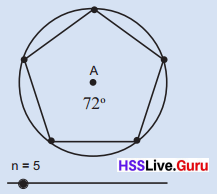Question 1.
How many squares are there in the picture? How many matchsticks are used to make it? How many matchsticks are needed to make five squares like this? In what different ways can we state relation between the number of squares and number of matchsticks?
Write them using letters.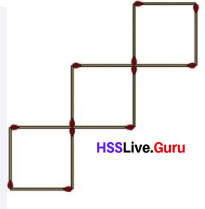Total number of matchsticks used = Number of squares in the figure × Number of matchsticks used in a square.
Number of matchsticks used to make 5 square = 20.

Explanation:
Number of squares in the figure = 3.
Number of matchsticks used in a square = 4.
=> Total number of matchsticks used = Number of squares in the figure × Number of matchsticks used in a square
= 3 × 4
= 12.
Number of squares needed to make = 5.
Number of matchsticks used to make 5 square = Number of squares needed to make × Number of matchsticks used in a square
= 5 × 4
= 20.Question 2.
All children in the school bought pens from the co-operative store, at 5 rupees each. Write in the table, how much the children of various classes paid.In what all ways can we say the relation between the number of children and the amount they paid? Write them using letters.
Total amount 6A childen paid = 170 rupees.
Total amount 6B childen paid = 160 rupees.
Total amount 6C childen paid = 180 rupees.

Explanation:
Number of children 6A = 34.
Number of children 6B = 32.
Number of children 6C = 36.
Cost of each pen children bought = 5 rupees.
Total amount 6A childen paid = Number of children 6A × Cost of each pen children bought
= 34 × 5
= 170 rupees.
Total amount 6B childen paid = Number of children 6A × Cost of each pen children bought
= 32 × 5
= 160 rupees.
Total amount 6C childen paid = Number of children 6A × Cost of each pen children bought
= 36 × 5
= 180 rupees.

Question 3.
What is the perimeter of a square of side 5 centimetres. What about a square of perimeter 6 centimetres? In what all ways can we say the relation between the length of a side and perimetre of a square? Write all these using letters.
Perimeter of a square (p)= 4 × Side of the square (s)

Explanation:
Side of the square (s)= 5 cm.
Perimeter of a square (p)= 4 × Side of the square (s)
= 4 × 5
= 20 cm.
Perimeter of a square (p) = 6 cm.
Perimeter of a square (p)= 4 × Side of the square (s)
=> 6 = 4 × Side of the square (s)
=> 6 ÷ 4 = Side of the square (s)
=> 1.5 cm = Side of the square (s)

Question 4.
How much money does 5 ten rupee notes make? What about 7 ten rupee notes? In what all ways can we say the number of ten rupee notes and the total amount? Using t to denote the number of ten rupee notes and a to denote the total amount, in what all ways can we write this relation?
Total amount 5 ten rupee notes make (a) = 50 rupees.
Total amount 7 ten rupee notes make (a) = 70 rupees.

Explanation:
One rupee note = 1 rupees.
Ten rupee note (t) = 10 rupees.
Total amount 5 ten rupee notes make (a) = 5 × Ten rupee note (t)
= 5 × 10
= 50 rupees.
Total amount 7 ten rupee notes make (a) = 7 × Ten rupee note (t)
= 7 × 10
= 70 rupees.

Multiplication again

What is the area of a rectangle of length 5 centimetres and breadth 3 centimetres?
How about a rectangle of length 5$$\frac{1}{2}$$ centimetres and breadth 3$$\frac{1}{4}$$ metres?
Whatever be the length and breadth, area is their product, right?
How do we write this using letters?

Taking the length as l centimetres, breadth as b centimetres and area as a square centimetres,
a = l × b = lb
See how we have omitted the multiplication sign here also.

Like this, the volume of a rectangular block is the product of its length, breadth and height.
This also we can write using letters. Taking length as l centimetres, breadth as b centimetres, height as h centimetres and volume as v cubic centimetres, we can write
v = lbhTextbook Page No. 157

Using the angles drawn in changing angle, we can draw a nice picture.
First change the values of slider n from 0 to 360. Use Circle with Centre through Point to draw a circle centred at A’ through A. Right click on the circle and choose trace on.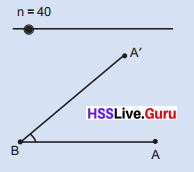Now right click on the slider and choose Animation on. Don’t you get a picture like this?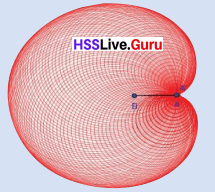Question 1.
What is the total price of 5 pens, each of price 8 rupees? What is the price of 10 notebooks, each of price 12 rupees?

i. In what all ways can we say the relation between the price of something, the number bought and the total price?
Total price (t) = Cost of each pen × Number of pens = 40 rupees.
Total price (t) = Cost of each notebook × Number of notebooks = 120 rupees.

Explanation:
Cost of each pen (p) = 8 rupees.
Number of pens (n) = 5.
Total price (t) = Cost of each pen × Number of pens
= 8 × 5
= 40 rupees.
Cost of each notebook (p) = 12 rupees.
Number of notebooks (n) = 10.
Total price (t) = Cost of each notebook × Number of notebooks
= 12 × 10
= 120 rupees.

ii. Taking the price of an article as p, their number as n and the total price as t, in what all ways can we write the relations between p, n and t ?
Total price of the articles (t) = Cost of each article (p) × Number of articles (n)

Explanation:
Total price of the articles (t) = Cost of each article (p) × Number of articles (n)
=> Total price of the articles (t) – Cost of each article (p)= Number of articles (n)
=> Total price of the articles (t) ÷ Number of articles (n) = Cost of each article (p)

Question 2.
One litre of kerosene weighs 800 grams.

i. What is the weight of 2 litres of keroscene?
Total weigh of kerosene = 1,600 liters.

Explanation:
Weigh of one litre of kerosene = 800 grams.
Number of liters of kerosene = 2.
Total weigh of kerosene = Weigh of one litre of kerosene × Number of liters of kerosene
= 800 × 2
= 1,600 liters.

ii. What is the weight of $$\frac{1}{2}$$ litre of keroscene?
Total weigh of kerosene = 400 liters.

Explanation:
Weigh of one litre of kerosene = 800 grams.
Number of liters of kerosene = $$\frac{1}{2}$$
Total weigh of kerosene = Weigh of one litre of kerosene × Number of liters of kerosene
= 800 × $$\frac{1}{2}$$
= 400 liters.

iii. What is the weight of 1 millilitre of keroscene?
Weight of 1 millilitre of keroscene = 0.8.

Explanation:
Weigh of one litre of kerosene = 800 grams.
1 milliliters of kerosene = ??
Conversation:
1 liter = 1000 milliliters.
=> ?? = 1 milliliter
=> 1 × 1 = ?? × 1000
=> 1 ÷ 1000 = ??
=> 800 ÷ 1000 = ??
=> 0.8 milliliters= ??

iv. Taking the weight of v millilitres of kersone as w grams, write a relation between v and w.
Weight of millilitres of kersone (v) = Weight of kersone ÷ 1000.

Explanation:
Weight of millilitres of kersone = (v)
Weight of kersone = w grams.
Conversation:
1 liter = 1000 milliliters.
=> Weight of millilitres of kersone (v) = Weight of kersone ÷ 1000.Question 3.
One cubic centimetre of iron weighs 7.8 grams.

i. Taking the volume of an iron object as v cubic centimetres and weight w grams, write a relation between v and w.
Density = w/v.

Explanation:
Weigh of one cubic centimetre of iron = 7.8 grams.
volume of an iron object = v cubic centimetres.
Weight of iron = w grams.
Weight is the amount of matter an object contains, while volume is how much space it takes up.

ii. Taking the length, breadth and height of a rectangular block of iron as l, b, h and its weight as w, write a relation between l, b, h and w.
A relation between l, b, h and w is that we get to know the area of the rectangular block of iron.

Explanation:
Length of the rectangular block of iron = l.
Breadth of the rectangular block of iron = b.
Height of the rectangular block of iron = h.
Weight of the rectangular block of iron = w.
=> Area of four walls =

Sequence rule

Look at these numbers:
1, 1, 2, 3, 5, 8, ………….
Can you say what the next number is?
For any three consecutive numbers a, b, c of this sequence, we must have a + b = c
Now try writing some more numbers of this. It is called Fibonacci sequence.

Ravi has 3 ten rupee notes and a one rupee coin; Lissy has 5 ten rupee notes and a one rupee coin.
How much money does Ravi have?
And Lissy?
How did you calculate?
Similarly how much does 25 ten rupee notes and a one rupee coin make?
(10 × 25) + 1 = 251
In general, how much money does some ten rupee notes and a one rupee coin make?
We have to multiply the number of notes by 10 and add 1, right?

Let’s write it using letters.
Take the number of ten rupee notes as t.
So how much money is t ten rupee notes and a one rupee coin?
What about 8 ten rupee notes and 7 one rupee coins?
What is the general method to calculate the amount of money, some ten rupee notes and some one rupee coins make?
Multiply the number of notes by 10 and add the number of coins.
How do we write this using letters?
t ten rupee notes and c coins make 10t + c rupees.

Question 1.
How much money does 8 ten rupee notes and 2 five rupee notes make? What about 7 ten rupee notes and 4 five rupee notes?

i. How do we say the relation between the number of ten rupee notes, the number of five rupee notes and the total amount?
The relation between the number of ten rupee notes, the number of five rupee notes and the total amount is ten rupee notes are 4 times greater than five rupee notes.

Explanation:
Number of ten rupee notes = 8.
Number of five rupee notes = 2.
Total amount = (Number of ten rupee notes × 10) + (Number of five rupee notes × 2)
= (8 × 10) + (5 × 2)
= 80 + 10
= 90 rupees.
Number of seven rupee notes = 7.
Number of five rupee notes = 4.
Total amount = (Number of ten rupee notes × 10) + (Number of five rupee notes × 2)
= (7 × 7) + (5 × 4)
= 49 + 20
= 69 rupees.

ii. Taking the number of ten rupee notes as t, the number of five rupee notes as f and the total amount as a how do we write the relation between t, f and a?
The relation between t, f and a is a direct relationship.

Explanation:
Number of ten rupee notes = t.
Number of five rupee notes = f.
Total amount = a.
Total amount = (Number of ten rupee notes × 10) + (Number of five rupee notes × 5)
=> a = (t × 10) + (f × 5)

Question 2.
The price of a pen is 7 rupees and the price of a notebook is 12 rupees.

i. What is the total price of 5 pens and 6 notebooks?
Total price of pens = 35 rupees.
Total price of notebooks = 72 rupees.

Explanation:
Price of a pen = 7 rupees.
Number of pens = 5.
Total price of pens = Price of a pen × Number of pens
= 7 × 5
= 35 rupees.
Price of a notebook = 12 rupees.
Number of notebooks = 6.
Total price of notebooks = Price of a notebook × Number of notebooks
= 12 × 6
= 72 rupees.

ii. What about 12 pens and 7 notebooks?
Total price of pens = 84 rupees.
Total price of notebooks = 84 rupees.

Explanation:
Price of a pen = 7 rupees.
Number of pens = 12.
Total price of pens = Price of a pen × Number of pens
= 7 × 12
= 84 rupees.
Price of a notebook = 12 rupees.
Number of notebooks = 7.
Total price of notebooks = Price of a notebook × Number of notebooks
= 12 × 7
= 84 rupees.

iii. What is the relation between the number of pens, the number of books and the total price?
The number of pens, the number of books and the total price is a direct relationship.

Explanation:
The number of pens, the number of books and the total price is a direct relationship as in number of pens increases the total price increases and same with the number of books.

iv. Taking the numbers of pens as p, the number of notebooks as n and total price as t, how do we write the relation between them?
The relation between them is that if any one increases among them automaticaaly the other one increases.

Explanation:
Numbers of pens = p.
Number of notebooks = n.
Total price = t.
If number of pens increases the total price of pens will increase and same with the number of notebooks.Question 3.
What length of wire is neeed to make a triangle of sides 10 centimetres each? To make a square of side 10 centimetres?

i. What is the total length needed to make 5 such triangles and 6 such squares?
Total length of wire is needed to make triangles = 50 cm.
Total length of wire is needed to make squares = 60 cm.

Explanation:
Length of wire is needed to make a triangle = 10 centimetres each.
Number of triangles = 5.
Total length of wire is needed = Length of wire is needed to make a triangle × Number of triangles
= 10 × 5
= 50 cm.
Length of wire is needed to make a square = 10 centimetres each.
Number of squares= 6.
Total length of wire is needed = Length of wire is needed to make a square × Number of squares
= 10 × 6
= 60 cm.

ii. What about 4 triangles and 3 squares?
Total length of wire is needed to make triangles = 40 cm.
Total length of wire is needed to make squares = 30 cm.

Explanation:
Length of wire is needed to make a triangle = 10 centimetres each.
Number of triangles = 4.
Total length of wire is needed = Length of wire is needed to make a triangle × Number of triangles
= 10 × 4
= 40 cm.
Length of wire is needed to make a square = 10 centimetres each.
Number of squares= 3.
Total length of wire is needed = Length of wire is needed to make a square × Number of squares
= 10 × 3
= 30 cm.

iii. What is the relation between the number of triangles, the number of squares and the total length of wire?
The number of triangles, the number of squares and the total length of wire is direct relationship.

Explanation:
The number of triangles, the number of squares and the total length of wire is that if number of triangles or squares increases the total length of them will increases.

iv. Taking the number of triangles as t, the number of squares as s and the total length as l , how do we write the relation between t, s and l?
The relation between t, s and l is that they are directly propotionate to eachother.

Explanation:
Number of triangles = t.
Number of squares = s.
Total length = l.
If number of triangles or squares increases the total length also increases.

Four friends went to buy pens and note books. The price of a pen is 8 rupees and the price of a notebook is 12 rupees. The shopkeeper calculated like this:
Price of 4 pens is 8 × 4 = 32 rupees
Price of 4 notebooks is 12 × 4 = 48 rupees
Total 80 rupees
The friends calculated like this:
The amount one has to spend is 8 + 12 = 20 rupees
Total expense 20 × 4 = 80 rupees
Another problem: we want to make a rectangle with eerkkil bits; of length 5$$\frac{1}{2}$$ centimetres and breadth 3$$\frac{1}{2}$$ centimetres. What is the total length of eerkkil needed?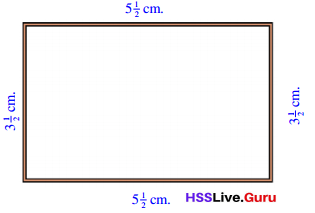We can calculate the total length as
5$$\frac{1}{2}$$ + 3$$\frac{1}{2}$$ + 5$$\frac{1}{2}$$ + 3$$\frac{1}{2}$$ = 18
Or, we can calculate it as two eerkkil bits of length 5$$\frac{1}{2}$$ centimetres and two of length 3$$\frac{1}{2}$$ centimetres;
(2 × 5$$\frac{1}{2}$$) + (2 × 3$$\frac{1}{2}$$) = 11 + 7 = 18There is a third way: take it as two eerkkil bits of length 5$$\frac{1}{2}$$ + 3$$\frac{1}{2}$$ centimetres:
(2 × 5$$\frac{1}{2}$$ + 3$$\frac{1}{2}$$) = 2 × 9 = 18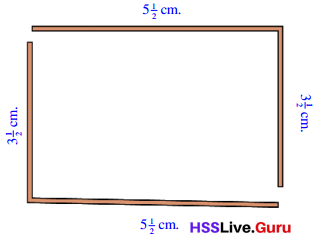Which is the easiest?

So if we take the length of a rectangle as l, breadth as b and perimeter as p, the relation between l, b andp can be written in various ways as
p = l + b + l + b
p = 2l + 2b
p = 2 (l + b)
Usually, the last one is the easiest to use.

For example, we can quickly calculate the perimeter of a rectangle of length 27 centimetres and breadth 43 centimetres as 2 × (27 + 43) = 140 centimetres.

Question 1.
There are 25 children in one room and 35 in another. 5 biscuits are to be given to each. How many biscuits are needed?
i. What if the number of children are 20 and 40?
Total number of biscuits needed = 300.

Explanation:
Number of children on one room = 20.
Number of children on another room = 40.
Number of biscuits given to each = 5.
Total number of biscuits needed = (Number of children on one room + Number of children on another room) × Number of biscuits given to each
= (20 + 40) × 5
= 60 × 5
= 300.

ii. If we take the number of children in the first room as f in the second room as s and the total number of biscuits as t, in what all ways can we write the relation between f s and t? What if each is given 6 biscuits instead of 5?
If there is a increase in the price of each biscuit the total number of biscuits needed also increases.

Explanation:
Number of children in the first room = f.
Number of children in the second room = s.
Total number of biscuits = t.
Total number of biscuits will be effected if there is a increase in the price of each biscuit increases.

iii. If the number of biscuits given to each is taken as b, in what all ways can we write the relation between f s, t and b?
t = (f + s) × b.

Explanation:
Number of biscuits given to each = b.
Number of children in the first room = f.
Number of children in the second room = s.
Total number of biscuits = t.
Total number of biscuits needed = (Number of children on one room + Number of children on another room) × Number of biscuits given to eachQuestion 2.
In the picture, M is the point right at the middle of AC.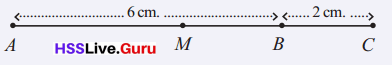What is the length of AM?
length of AM = 4 cm.

Explanation:
Length of AC = AB + BC
=> 6 + 2
= 8 cm.
M is the point right at the middle of AC.
=> Length of AM = Length of AC ÷ 2
=> 8 ÷ 2
= 4 cm.

i. If a 5 centimetre long line is extended by 4 centimetres, at what distance from an end point of the longer line is the point exactly at its middle?
Length of the line from an end point of the longer line is the point exactly at its middle =4.5 cm.

Explanation:
Length of long line = 5 cm.
Length of the line extended = 4 cm.
Total length of the line = Length of long line + Length of the line extended
= 5 + 4
= 9 cm
Length of the line from an end point of the longer line is the point exactly at its middle = Total length of the line ÷ 2
= 9 ÷ 2
= 4.5 cm.

ii. What if a 7$$\frac{1}{2}$$ centimetre long line is extended by 2$$\frac{1}{2}$$ centimetres?
Length of the line from an end point of the longer line is the point exactly at its middle = 5 cm.

Explanation:
Length of long line = 7$$\frac{1}{2}$$ cm.
Length of the line extended = 2$$\frac{1}{2}$$ cm.
Total length of the line = Length of long line + Length of the line extended
= 7$$\frac{1}{2}$$ + 2$$\frac{1}{2}$$
= {[(7 × 2) + 1] ÷ 2} + {[(2 × 2) + 1] ÷ 2}
= [(14 + 1) ÷ 2] + [(4 + 1) ÷ 2]
= $$\frac{15}{2}$$ + $$\frac{5}{2}$$
= (15 + 5) ÷ 2
= $$\frac{20}{2}$$
= 10 cm.
Length of the line from an end point of the longer line is the point exactly at its middle = Total length of the line ÷ 2
= 10 ÷ 2
= 5 cm.

iii. A line of length l centimetres is extended by e centimetres. The mid point of the long line, is m centimetres away from one of its end points. What is the relation between l, e and m?
There is a direct relationship between l, e and m.

Explanation:
Line of length = l centimetres.
Line of length is extended = e centimetres.
Mid point of the long line = m centimetres.
The relation between if line length increases the mid point distance to the end point also increases.

Question 3.
The length of a rectangle is 4 centimetres and its breadth is 3 centimetres. The length is increased by 2 centimetres to make a large rectangle.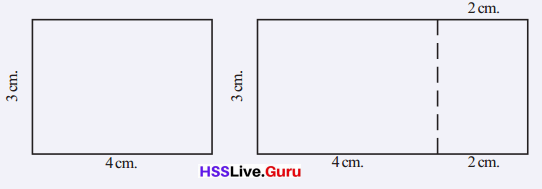i. What is the area of the large rectangle? If the length is increased by 3 centimetres, what is the area of the large rectangle?
Area of large rectangle = 42 square cm.

Explanation:
Length of the rectangle = 4 cm.
Breadth of the rectangle = 3 cm.
Area of rectangle = Length of the rectangle  × Breadth of the rectangle
= 4 × 3
= 12 square cm.
length is increased by 3 centimetres
=> Length of the rectangle = 4 cm + 3 cm = 7 cm.
Breadth of the rectangle = 3 cm + 3 cm = 6 cm.
Area of large rectangle = Length of the rectangle  × Breadth of the rectangle
= 7 × 6
= 42 square cm.

ii. Taking the length and breadth of the original rectangle as l centimetres and b centimetres, the increase in length as i centimetres and the area of the large rectangle as a square centimetres, in what all ways can we write the relation between l, b, i and a?